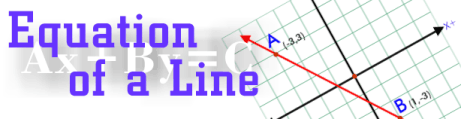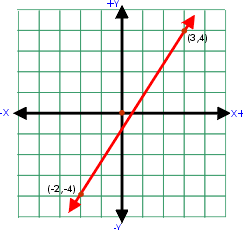The standard form for the equation of a line is Ax + By = C. A, B and C are real numbers and, x and y are variables. To find the equation of a linethis formula is used: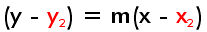Both x and y remain variables. The slope, m, will be a real number as well as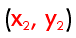.Recall the formula for a slope.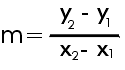Step 1: Find the slope of the line.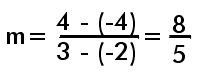Step 2: Substitute values into the formula.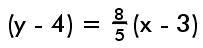Step 3: Use the distributive property.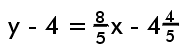Step 4: Use the addition property of equality to combinethe constants, and to place x and its coefficient across the equal sign.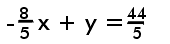Step 5: Multiply by the denominator to create integer coefficients.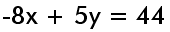practice the standard form equation